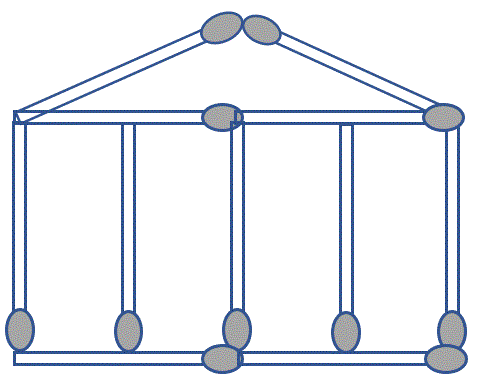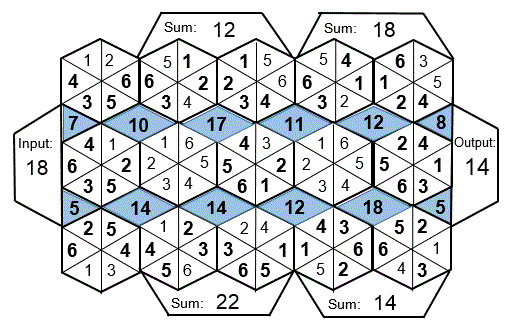Select Page

Each month, a new set of puzzles will be posted.  Come back next month for the solutions and a new set of puzzles, or subscribe to have them sent directly to you.

## MATCHSTICK Challenge

Here is a figure with 11 matchsticks. Move two matchsticks to make eleven squares (of any size). All matchsticks must be used.## MIND-Xpander (Circles in a Rectangle Puzzle – Level 2)

The diagram below shows two equal and touching circles completely inside a rectangle as shown below. The circles have the same radius, are not over-lapping and each touches two sides of the rectangle, as shown. What are the radii of the circles if the dimensions of the rectangle are 48cm by 54cm? Note: The diagram is not drawn to scale.## Feedback

There are more than one way of doing these puzzles and may well be more than one answer.  Please let me and others know what alternatives you find by commenting below.  We also welcome general comments on the subject and any feedback you'd like to give.

If you have a question that needs a response from me or you would like to contact me privately, please use the contact form.

## Get more puzzles!

If you've enjoyed doing the puzzles, consider ordering the books;

• Book One - 150+ of the best puzzles
• Book Two - 200+ with new originals and more of your favourites

Both in a handy pocket sized format.   Click here for full details.

## HEXAGON-numeric puzzle (Level 1)

Fit the numbers 1 – 6 in each hexagon and where the hexagon segments touch each other, the numbers in these segments will be the same. No number can be repeated in a hexagon.

## HEXAGON-numeric puzzle (Level 3)

Fit the numbers 1 – 6 in each hexagon and where the hexagon sides touch each other, the numbers in these 2 segments will be the same. No number can be repeated in a hexagon. The numbers in the shaded areas are the sum of the numbers in the 2 or 4 segments that they side with. The numbers in the ‘Input’ and ‘Output’ boxes are the sum of the numbers in the 3 triangular segments that they side with. The numbers in the ‘Sum’ boxes are the sum of the numbers in the 4 segments that they side with. Solve the puzzle and find the Output’ number (?).